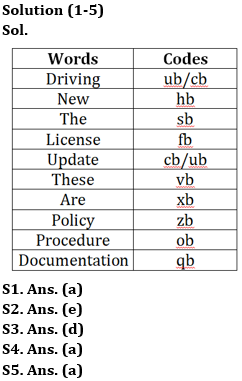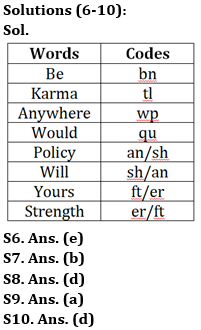Latest Banking jobs   »

# Reasoning Ability Quiz For Bank Foundation 2023 -23rd April

Directions (1-5): Answer the following questions based on the information given below.
In a certain code of language,
“The new driving license update” is coded as “hb sb cb ub fb”
“These are policy license” is coded as “fb xb zb vb”
‘’New are procedure” is coded as “xb ob hb”
“The policy documentation” is coded as “qb sb zb”

Q1. What is the code for the word ‘these’?
(a) vb
(b) xb
(c) zb
(d) fb
(e) None of these

Q2. What is the code for the word ‘update’?
(a) ub
(b) cb
(c) hb
(d) sb
(e) Can’t be determined

Q3. The code ‘zb’ represents which of the following word?
(a) These
(b) Documentation
(d) Policy
(e) None of these

Q4. What is the code for ‘are’?
(a) xb
(b) fb
(c) ob
(d) hb
(e) None of these

Q5. What is the code for the word ‘Documentation’?
(a) qb
(b) sb
(c) zb
(d) None of these
(e) Can’t be determined

Direction (6-10): Study the following information and answer the given questions.
In coded language,
“Karma would be anywhere” is coded as “wp bn qu tl”
“Anywhere policy will be” is coded as “an wp bn sh”
“Karma be yours strength” is coded as “tl bn ft er”

Q6. What is the code for the word ‘strength’?
(a) ft
(b) er
(c) bn
(d) tl
(e) Either ft or er

Q7. If ‘will please’ is coded as ‘sh pr’ then what can be the possible code for ‘policy care’ in the coded language?
(a) an bn
(b) an ut
(c) ut wp
(d) ut tl
(e) sa pz

Q8. Which among the following word is coded as ‘bn’?
(a) Anywhere
(b) Karma
(c) Would
(d) Be
(e) None of the above

Q9. What is the code for the word ‘anywhere’?
(a) wp
(b) qu
(c) tl
(d) bn
(e) None of the above

Q10. What is the code for the word ‘Yours’?
(a) ft
(b) er
(c) sh
(d) Either ft or er
(e) None of the above

Directions (11-13): Study the information carefully and answer the questions given below.
Six persons M, N, O, P, Q, and S are of different weight. P is heavier than N. M is lighter than S. Q is heavier than N. M is not lighter than P and Q. S is not heaviest, and Q is not second lightest. The weight of heaviest is 70kg.

Q11. Who among the following person is third heaviest?
(a) M
(b) S
(c) P
(d) Q
(e) Can’t be determined

Q12. Who among the following is the lightest person?
(a) M
(b) N
(c) Q
(d) P
(e) None of these

Q13. If weight of M is 67kg what may be the weight of S?
(a) 60kg
(b) 63kg
(c) 61kg
(d) 69kg
(e) None of these

Q14. If in the number 39475132, 2 is multiply to each of the digit which is less than 4 and 3 is subtracted from each of the digit which is more than 4 and equal to 4 then how many digits are repeating in the number thus formed?
(a) None
(b) Four
(c) One
(d) Three
(e) Two

Q15. If all the letters in the word CONSTITUTION are arranged in alphabetical order from left to right, then how many alphabets remains same in their position?
(a) Three
(b) One
(c) None
(d) Two
(e) four

SolutionsSolution (11-13):
Sol. O (70kg) > S > M > Q > P > N

S11. Ans. (a)
S12. Ans. (b)
S13. Ans. (d)

S14. Ans. (d)

S15. Ans. (d)
Sol. Original Word- CONSTITUTION
After operation- CIINNOOSTTTU## FAQs

### What is the selection process of the Bank Clerk?

The selection process of the Bank Clerk is Prelims & Mains.

#### Congratulations!Union Budget 2023-24: Free PDF